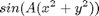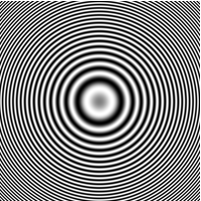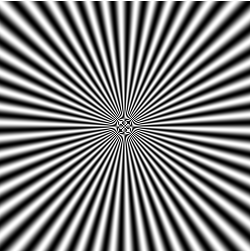# Synthesizing images using simple equations

In 1988, Gerard Holzmann of AT&T Bell Labs (back when there was such a thing) published a fun book called Beyond Photography: The Digital Darkroom (. The book shows how some simple math on pixel values and coordinates can transform images in fascinating ways.

In the first part of the book, Holzmann shows how to synthesize interesting images using functions of both Cartesian and polar coordinates. Let's see how to do that in MATLAB.

MATLAB functions featured: meshgrid, cart2pol

Image Processing Toolbox functions featured: imshow

## Contents

The MATLAB function meshgrid is extremely useful for computing a function of two Cartesian coordinates, and you can make some interesting images this way.

meshgrid is kind of hard to explain in words. It's easier to just look at what it does.

x = 1:3;
y = 10:14;
[xx, yy] = meshgrid(x, y)
xx =

1     2     3
1     2     3
1     2     3
1     2     3
1     2     3

yy =

10    10    10
11    11    11
12    12    12
13    13    13
14    14    14



## Concentric rings

Let's make an image out of the equation:x = linspace(-pi, pi, 201);
% If you pass meshgrid only one vector, it uses that vector for both the x
% and the y coordinates.
[xx,yy] = meshgrid(x);
A = 10;
I = sin(A*(xx.^2 + yy.^2));

% Specify the range -1 to 1 when displaying the image.
imshow(I, [-1 1])## Using polar coordinates

If you want to construct an image from a function of polar coordinates, use cart2pol in conjunction with meshgrid.

(The interesting patterns you see in the center of the image below result from ''aliasing,'' but that's a topic for another day.)

[xx,yy] = meshgrid(-125:125);
[theta,R] = cart2pol(xx,yy);
I = sin(50*theta);
imshow(I, [-1 1])Published with MATLAB® 7.1

|